# Project Euler – Problem 64 Solution

Check out my new course Learn you some Lambda best practice for great good! and learn the best practices for performance, cost, security, resilience, observability and scalability.

#### Problem

All square roots are periodic when written as continued fractions and can be written in the form: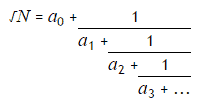For example, let us consider ?23: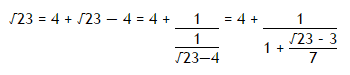If we continue we would get the following expansion: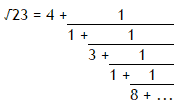The process can be summarised as follows: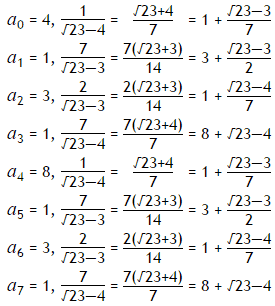It can be seen that the sequence is repeating. For conciseness, we use the notation ?23 = [4;(1,3,1,8)], to indicate that the block (1,3,1,8) repeats indefinitely.

The first ten continued fraction representations of (irrational) square roots are:

?2=[1;(2)], period=1

?3=[1;(1,2)], period=2

?5=[2;(4)], period=1

?6=[2;(2,4)], period=2

?7=[2;(1,1,1,4)], period=4

?8=[2;(1,4)], period=2

?10=[3;(6)], period=1

?11=[3;(3,6)], period=2

?12= [3;(2,6)], period=2

?13=[3;(1,1,1,1,6)], period=5

Exactly four continued fractions, for N <= 13, have an odd period.

How many continued fractions for N <= 10000 have an odd period?

#### Solution

(see full solution here).

Based on the algorithm on continued fractions from Wikipedia, we can implement the expansion algorithm as: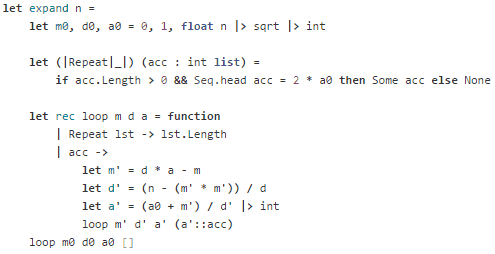The algorithm can also terminate on ai when ai = 2 a0, which is easier to implement.

which corresponds to the termination condition we have in the Repeat active pattern (which also checks if the accumulator is empty):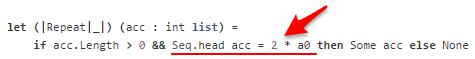Also, this algorithm doesn’t work on numbers that are perfect squares, i.e. 4, 9, 16, … hence we need to exclude them when searching for our answer.

Here’s the solution in full: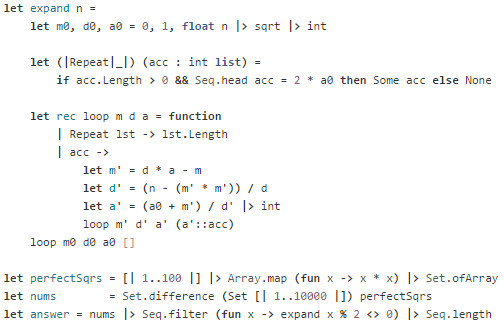This solution took 92ms to execute on my machine.Hi, I’m Yan. I’m an AWS Serverless Hero and the author of Production-Ready Serverless.

I specialise in rapidly transitioning teams to serverless and building production-ready services on AWS.

Are you struggling with serverless or need guidance on best practices? Do you want someone to review your architecture and help you avoid costly mistakes down the line? Whatever the case, I’m here to help.Check out my new course, Learn you some Lambda best practice for great good! In this course, you will learn best practices for working with AWS Lambda in terms of performance, cost, security, scalability, resilience and observability. Enrol now and enjoy a special preorder price of £9.99 (~\$13).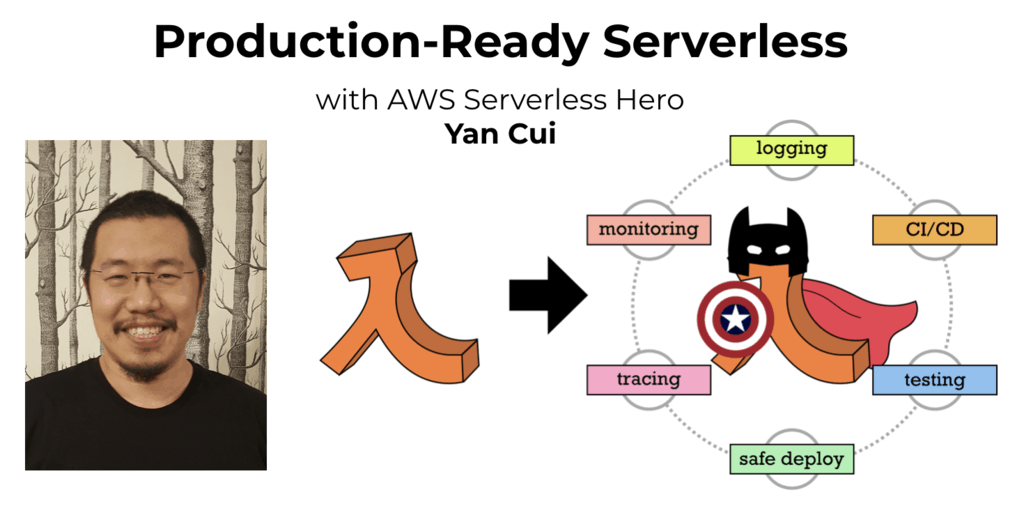Are you working with Serverless and looking for expert training to level-up your skills? Or are you looking for a solid foundation to start from? Look no further, register for my Production-Ready Serverless workshop to learn how to build production-grade Serverless applications!